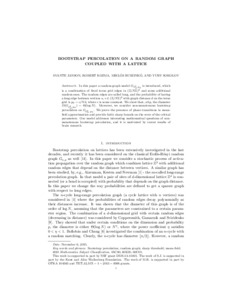REAL

# Bootstrap percolation on a random graph coupled with a lattice

Janson, Svante and Kozma, Róbert and Ruszinkó, Miklós and Sokolov, Yury (2016) Bootstrap percolation on a random graph coupled with a lattice. ELECTRONIC JOURNAL OF COMBINATORICS. ISSN 1097-1440 (In Press)Preview
Text
sj305.pdf

In this paper a random graph model $G_{\mathbb{Z}^2_N,p_d}$ is introduced, which is a combination of fixed torus grid edges in $(\mathbb{Z}/N \mathbb{Z})^2$ and some additional random ones. The random edges are called long, and the probability of having a long edge between vertices $u,v\in(\mathbb{Z}/N \mathbb{Z})^2$ with graph distance $d$ on the torus grid is $p_d=c/Nd$, where $c$ is some constant. We show that, {\em whp}, the diameter $D(G_{\mathbb{Z}^2_N,p_d})=\Theta (\log N)$. Moreover, we consider non-monotonous bootstrap percolation on $G_{\mathbb{Z}^2_N,p_d}$. We prove the presence of phase transitions in mean-field approximation and provide fairly sharp bounds on the error of the critical parameters. Our model addresses interesting mathematical questions of non-monotonous bootstrap percolation, and it is motivated by recent results of brain research.Edit Item